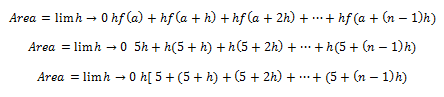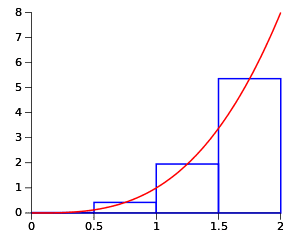# Definite Integral by Definition

## Homework Statement

Let A be the area of the region that lies under the graph of f(x) = 2x 2 + 5 between x = 0 and x = 4. Find an expression for A using n rectangles. Then evaluate this expression.

h= (4/n)

## The Attempt at a Solution[/B]
The problem I am having is I do not know how to simplify this limit further after factoring out the h.

Last edited by a moderator:

BvU
Homework Helper
##f(a+h)## is not ##5+h## but ##2(a+h)^2+5## !?

Aren't you supposed to find ##A(n)## ?

##f(a+h)## is not ##5+h## but ##2(a+h)^2+5## !?

Aren't you supposed to find ##A(n)## ?

You are right about 2(a+h)^2+5. So you would suggest to convert this limit all to "n" and evaluate the limit as n ---> infinity?

BvU
Homework Helper
If you have faithfully rendered the problem statement, the answer is an expression. Something like ##\displaystyle A = \sum_{i=1}^n \ ... \ ##

The way I read it you want to work out e.g. the middle riemann sumRay Vickson
Homework Helper
Dearly Missed

## Homework Statement

Let A be the area of the region that lies under the graph of f(x) = 2x 2 + 5 between x = 0 and x = 4. Find an expression for A using n rectangles. Then evaluate this expression.

h= (4/n)

## The Attempt at a Solution[/B]
The problem I am having is I do not know how to simplify this limit further after factoring out the h.

I cannot read your formula for f(x). Is it ##f(x) = 2x^2 + 5##? ##f(x) = (2x)^2 + 5##? Something else? If it really does involve the second power (and if you don't want to use either LaTeX or the formula template in the top green panel, then you need to use "^" instead, as in 2 x^2 + 5 or (2x)^2 + 5.

BvU
Homework Helper
Well, the book answer helps you out, RayRay Vickson
Homework Helper
Dearly Missed
Well, the book answer helps you out, RayWell, of course I can guess what the OP means, but the whole point is that I should not need to. He/she needs to adhere to the standard notation; that's why it was invented.

BvU